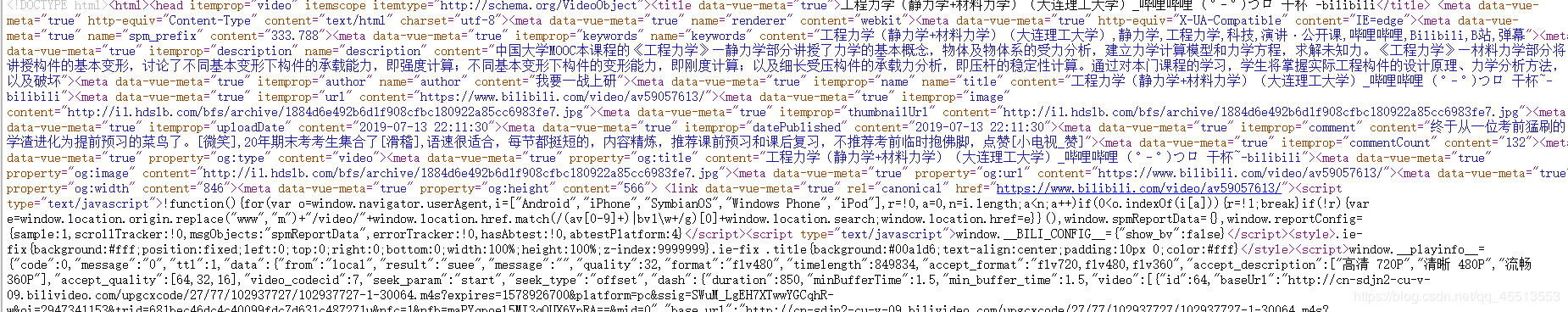# Python实现bilibili时间长度查询的示例代码```import requests
import re
import pygame
import time

header = {'User-Agent': 'Mozilla/5.0 (Windows NT 6.1; Win64; x64) AppleWebKit/537.36 (KHTML, like Gecko) '
'Chrome/74.0.3729.157 ''Safari/537.36 '}

class Bili(object):
def __init__(self, url):
self.url = url
self.max_page = 0
self.the_time = list()
self.sum_time = 0
self.b_url = 'https://www.bilibili.com/video/av' + re.search(r'av(\d+)', url).group(1) + '?p='

@staticmethod
def get_html(acp_url):
try:
r.raise_for_status()
r.encoding = r.apparent_encoding
return r.text
except Exception as result:
print("get_html:", result)
return ""

def parse_max_page(self):
self.max_page = int(re.findall(r'videos":(.+?),', self.get_html(self.url)))

def parse_time(self, se_url):
text = self.get_html(se_url)
single_time = re.findall(r'"timelength":(\d+),', text)[:-3]
return single_time

def get_time(self):
for i in range(self.max_page + 1):
s_url = self.b_url + str(i)
ret = self.parse_time(s_url)
self.the_time.append(ret)
print("第%d页 time=%s" % (i, ret))
for each in self.the_time:
self.sum_time += int(each)
print("sum_time:%.3s h" % str(self.sum_time / 60))

def main(self):
self.parse_max_page()
self.get_time()

if __name__ == '__main__':
i_url = input("请输入链接地址[要以+结尾]：")
Bili(i_url).main()

```

#### 您可能感兴趣的文章:

• 本文相关：
• php中获得视频时间总长度的另一种方法
• 用php读取flv文件的播放时间长度
• 用vbscript实现修改屏幕保护的等待时间长度
• 如何基于python操作excel并获取内容
• pandas统计重复的列里面的值方法
• python遍历小写英文字母的方法
• python中pip安装非pypi官网第三方库的方法
• 在django中创建第一个静态视图
• python版学生管理系统
• python字典一键多值实例代码分享
• python数据结构之顺序表的实现代码示例
• 基于python实现的扫雷游戏实例代码
• 解决python opencv无法显示图片的问题
• 免责声明 - 关于我们 - 联系我们 - 广告联系 - 友情链接 - 帮助中心 - 频道导航